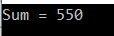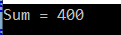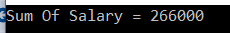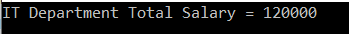# Linq Sum Method in C# with Examples

In this article, I am going to discuss the Linq Sum Method in C# with examples. Please read our previous article before proceeding to this article where we discussed the basics of Linq Aggregate Functions in C# with some examples. As part of this article, we are going to discuss the following pointers.

1. What is Linq Sum Method in C#?
2. Multiple examples using both Method and Query syntax.
##### What is Linq Sum Method in C#?

The Linq Sum() Method belongs to the category of Aggregate Functions. The Linq Sum method in C# is used to calculates the total or sum of numeric values in the collection. Let us understand the Sum() method with some examples.

##### Example1:

The following example calculates the sum of all integers present in the collection.

```using System;
using System.Linq;
namespace LINQDemo
{
class Program
{
static void Main(string[] args)
{
int[] intNumbers = new int[] { 10, 30, 50, 40, 60, 20, 70, 90, 80, 100 };

//Using Method Syntax
int MSTotal = intNumbers.Sum();

//Using Query Syntax
int QSTotal = (from num in intNumbers
select num).Sum();

Console.WriteLine("Sum = " + QSTotal);

}
}
}```

Output:Note: We don’t have any operator called sum in Linq query syntax. So here we need to use the mixed syntax.

##### Example2: Sum Method with filter

Now we need to calculate the sum of all numbers which is greater than 50.

```using System;
using System.Linq;
namespace LINQDemo
{
class Program
{
static void Main(string[] args)
{
int[] intNumbers = new int[] { 10, 30, 50, 40, 60, 20, 70, 90, 80, 100 };

//Using Method Syntax
int MSTotal = intNumbers.Where(num => num > 50).Sum();

//Using Query Syntax
int QSTotal = (from num in intNumbers
where num > 50
select num).Sum();

Console.WriteLine("Sum = " + QSTotal);

}
}
}```

Output:##### Example3: Sum Method with Predicate

Instead of using the where method to filter the data, you can also use the other overloaded version of the Sum method which takes a Predicate and within that predicate, you can write the logic to filter the data as shown in the below example.

```using System;
using System.Linq;
namespace LINQDemo
{
class Program
{
static void Main(string[] args)
{
int[] intNumbers = new int[] { 10, 30, 50, 40, 60, 20, 70, 90, 80, 100 };

//Using Method Syntax with a Predicate
int MSTotal = intNumbers.Sum(num => {
if (num > 50)
return num;
else
return 0;
});

Console.WriteLine("Sum = " + MSTotal);

}
}
}```

Output:##### Working with Complex Type:

We are going to work with the following Employee class.

```using System.Collections.Generic;
namespace LINQDemo
{
public class Employee
{
public int ID { get; set; }
public string Name { get; set; }
public int Salary { get; set; }
public string Department { get; set; }

public static List<Employee> GetAllEmployees()
{
List<Employee> listStudents = new List<Employee>()
{
new Employee{ID= 101,Name = "Preety", Salary = 10000, Department = "IT"},
new Employee{ID= 102,Name = "Priyanka", Salary = 15000, Department = "Sales"},
new Employee{ID= 103,Name = "James", Salary = 50000, Department = "Sales"},
new Employee{ID= 104,Name = "Hina", Salary = 20000, Department = "IT"},
new Employee{ID= 105,Name = "Anurag", Salary = 30000, Department = "IT"},
new Employee{ID= 106,Name = "Sara", Salary = 25000, Department = "IT"},
new Employee{ID= 107,Name = "Pranaya", Salary = 35000, Department = "IT"},
new Employee{ID= 108,Name = "Manoj", Salary = 11000, Department = "Sales"},
new Employee{ID= 109,Name = "Sam", Salary = 45000, Department = "Sales"},
new Employee{ID= 110,Name = "Saurav", Salary = 25000, Department = "Sales"}
};

return listStudents;
}
}
}```

This is a very simple Employee class with having four properties such as ID, Name, Salary, and Department. We also create one method i.e. GetAllEmployees() which will return the list of all the employees.

##### Example4:

The following example calculates the Sum of Salaries of all the employees.

```using System;
using System.Linq;
namespace LINQDemo
{
class Program
{
static void Main(string[] args)
{
//Using Method Syntax
var TotalSalaryMS = Employee.GetAllEmployees()
.Sum(emp => emp.Salary);

//Using Query Syntax
var TotalSalaryQS = (from emp in Employee.GetAllEmployees()
select emp).Sum(e => e.Salary);

Console.WriteLine("Sum Of Salary = " + TotalSalaryMS);

}
}
}```

Output:##### Example5:

The following example calculates the sum of the salary of all the employees who belong to the IT department.

```using System;
using System.Linq;
namespace LINQDemo
{
class Program
{
static void Main(string[] args)
{
//Using Method Syntax
var TotalSalaryMS = Employee.GetAllEmployees()
.Where(emp => emp.Department == "IT")
.Sum(emp => emp.Salary);

//Using Query Syntax
var TotalSalaryQS = (from emp in Employee.GetAllEmployees()
where emp.Department == "IT"
select emp).Sum(e => e.Salary);

Console.WriteLine("IT Department Total Salary = " + TotalSalaryQS);

}
}
}```

Output:##### Example6:

Let’s rewrite the previous example using custom predicate.

```using System;
using System.Linq;
namespace LINQDemo
{
class Program
{
static void Main(string[] args)
{
//Using Method Syntax and Predicate
var TotalSalaryMS = Employee.GetAllEmployees()
.Sum(emp => {
if (emp.Department == "IT")
return emp.Salary;
else
return 0;
});

Console.WriteLine("IT Department Total Salary = " + TotalSalaryMS);